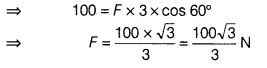# If the same amount of force is applied to move the cart to a distance of 5 m, then calculate the work done

If a boy is pulling a cart with a string which makes an angle of 60° with the horizontal to move the cart. Then, the network done in moving the cart to a distance of 3 m is 100 J. Now, if the same amount of force is applied to move the cart to a distance of 5 m, then calculate the work done.

Given, θ = 60°, W = 100 J, s = 3m
As we know,
Work done, W = Force (F) × displacement (s) × cos θNow, s = 5m